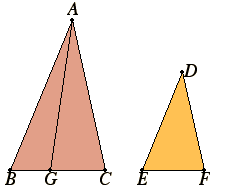# Proposition 19

Similar triangles are to one another in the duplicate ratio of the corresponding sides.
V.Def.11

Let ABC and DEF be similar triangles having the angle at B equal to the angle at E, and such that AB is to BC as DE is to EF, so that BC corresponds to EF.

I say that the triangle ABC has to the triangle DEF a ratio duplicate of that which BC has to EF.VI.11

Take a third proportional BG to BC and EF so that BC is to EF as EF is to BG, and join AG.

V.16

Since AB is to BC as DE is to EF, therefore, alternately, AB is to DE as BC is to EF.

V.11

But BC is to EF as EF is to BG, therefore also AB is to DE as EF is to BG.

Therefore in the triangles ABG and DEF the sides about the equal angles are reciprocally proportional.

VI.15

But those triangles which have one angle equal to one angle, and in which the sides about the equal angles are reciprocally proportional, are equal. Therefore the triangle ABG equals the triangle DEF.

V.Def.9

Now since BC is to EF as EF is to BG, and, if three straight lines are proportional, the first has to the third a ratio duplicate of that which it has to the second, therefore BC has to BG a ratio duplicate of that which BC has to EF.

But BC is to BG as the triangle ABC is to the triangle ABG, therefore the triangle ABC also has to the triangle ABG a ratio duplicate of that which BC has to EF.

V.7

But the triangle ABG equals the triangle DEF, therefore the triangle ABC also has to the triangle DEF a ratio duplicate of that which BC has to EF.

Therefore, similar triangles are to one another in the duplicate ratio of the corresponding sides.

Q.E.D.

# Corollary

From this it is clear that if three straight lines are proportional, then the first is to the third as the figure described on the first is to that which is similar and similarly described on the second.

## Guide

This proposition is used in the proof of the next one, and the corollary is used in the proofs of VI.22, VI.31, and X.6.